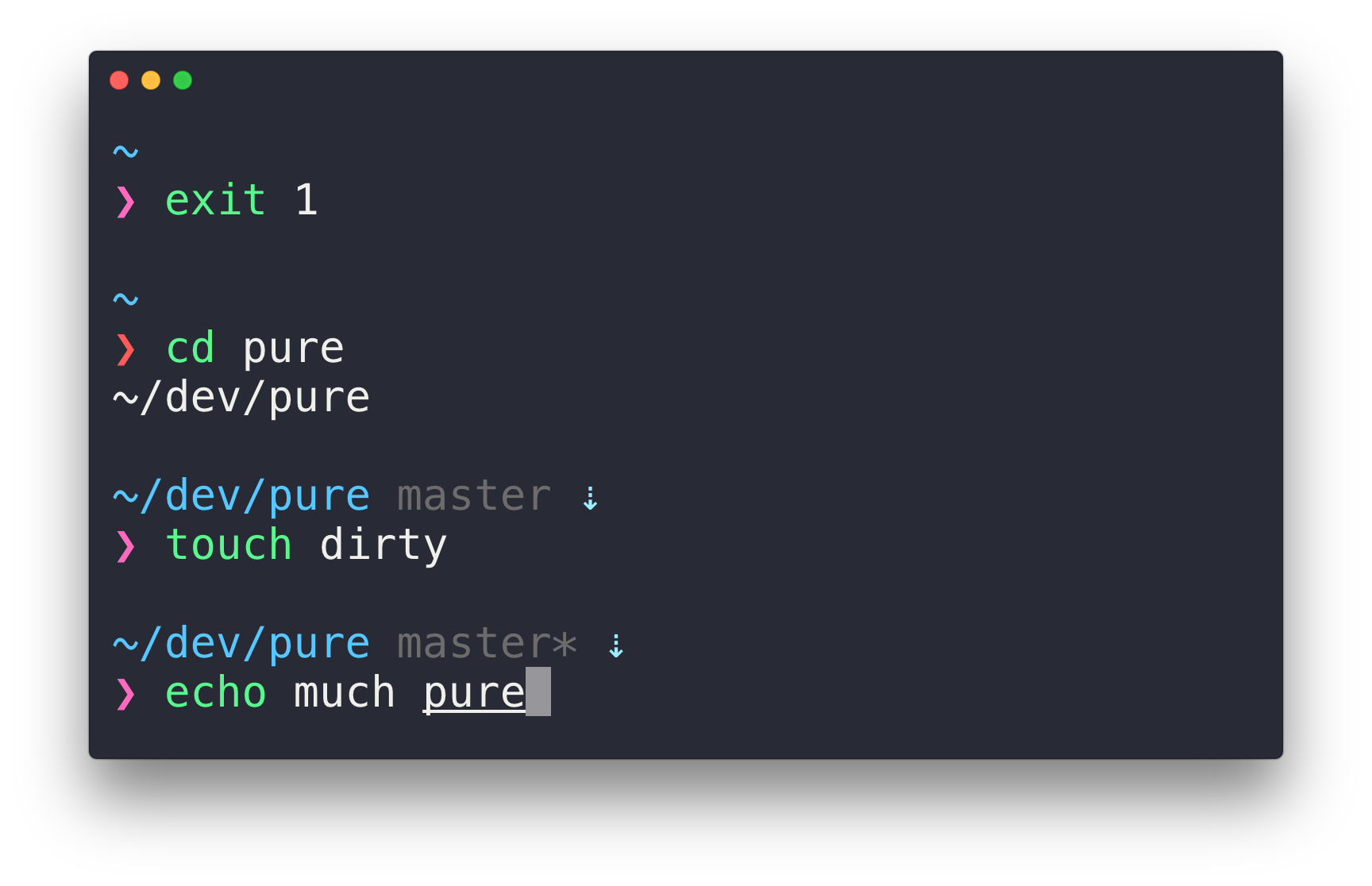Mathematica: Faster Multinormal Sampling with Different Covariance Matrices

Let's say we are given a set of different covariance matrices. We wish to draw a single sample of the multivariate normal distribution from each of these covariance matrices.

Assuming the mean vector is zero, we would code this for a single draw from a single covariance matrix sigma in Mathematica:

MultinormalDraw[sigma_] :=
RandomVariate[MultinormalDistribution[sigma]]

To demonstrate why this is slow, we will use a hundred randomly generated $200\times 200$ covariance matrices (that is, matrices that are both symmetric and positive definite):

Multivariable Epsilon-Delta proof example

Multivariable epsilon-delta proofs are generally harder than their single variable counterpart. The difficulty comes from the fact that we need to manipulate $|f(x,y) - L|$ into something of the form $\sqrt{(x-a)^2 + (y-b)^2}$, which is much harder to do than the simple $|x-a|$ with single variable proofs. This often requires several ingenious algebraic tricks that, at first don't seem to make much sense. Consider the following example.

Let $$f : \{(x,y)\in {\mathbb{R}}^2 : x^2 \ne y^2\} \to \mathbb{R}$$ be defined by

\begin{equation*} f(x,y) = \frac{x^3 - y^3}{x^2-y^2}. \end{equation*}

Prove that the limit

\begin{equation*} \lim_{(x,y)\to (a,a)} f(x,y) \end{equation*}

exists for all real $a \ne 0$.

Modern Portfolio Theory and Efficient Frontier

Suppose we are given two stocks, stock $$A$$ and stock $$B$$. We have \$$$10,000$$ we wish to invest. How much should we invest in each of these stocks?

Compute π using integrals

In our previous post, we looked at

\begin{equation*} I = {\int_{0}^{1}}{\frac{x^{4}(1-x)^{4}}{x^2+1}}{\,dx}. \end{equation*}

Let's now consider the generalized integral

\begin{equation*} I_n = {\int_{0}^{1}}{\frac{x^{4n}(1-x)^{4n}}{x^2+1}}{\,dx}. \end{equation*}

Prove using integration: π is not 22/7.

Consider the infamous definite integral

\begin{equation*} I = {\int_{0}^{1}}{\frac{x^4(1-x)^4}{x^2+1}}{\,dx}. \end{equation*}

A function whose inverse is equal to its derivative

Here's a problem: find a function $$f$$ whose inverse $$f^{-1}$$ is equal to its derivative $$f'$$.

Arch Linux post-install setup

Arch Linux is a very minimalistic distribution. After a fresh installation, many tasks need to be done to make the system usable.

A cool formula that plots itself

Take a look at this nice little inequality:

\begin{equation*} \frac12 < \left\lfloor \mathrm{mod} \left( \left\lfloor{\frac{y}{17}}\right\rfloor 2^{-17\lfloor x \rfloor - \mathrm{mod}(\lfloor y \rfloor, 17)}, 2 \right) \right\rfloor \end{equation*}

Install Android SDK via CLI on Ubuntu

Open source software is nice. It gives you the freedom to compile the software yourself, so you don't have to rely on somebody else's binary. To fully utilize this perk, you need to be able to compile the source.